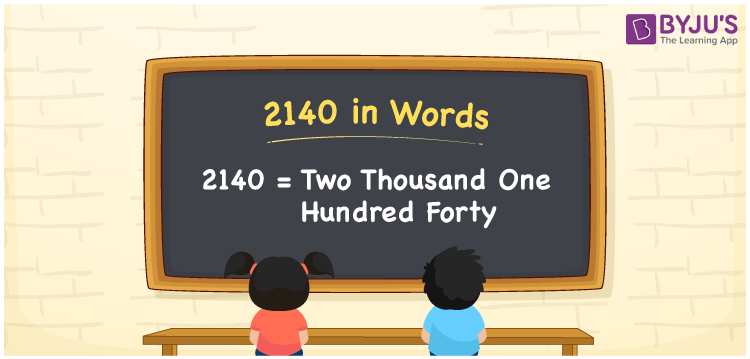# 2140 in Words

We can write 2140 in words as Two Thousand One Hundred Forty. For instance, Shweta purchased a handbag worth Rs. 2140, then you can say, “Shweta purchased a handbag worth Rupees Two Thousand One Hundred Forty”. 2140 is a cardinal number since it shows a definite amount. Let us understand in detail how to write the numerical name for 2140 with the help of a place value chart and its spelling in English in this article.

 2140 in Words Two Thousand One Hundred Forty Two Thousand One Hundred Forty in numerical form 2140

## 2140 in English Words

In Maths, we usually write numbers in words with the help of the English alphabet. Therefore, the number 2140 in English can be spelt as Two Thousand One Hundred Forty.## How to Write 2140 in Words?

In this section, we will learn the conversion of 2140 in words using a place value chart of 4 columns since 2140 is a four-digit number.

 Thousands Hundreds Tens Ones 2 1 4 0

Hence, we can write the expanded form as:

2 x Thousand + 1 x Hundred + 4 x Ten + 0 x One

= 2 x 1000 + 1 x 100 + 4 x 10 + 0 x 1

= 2000 + 100 + 40 + 0

= 2000 + 100 + 40

= 2140

= Two Thousand One Hundred Forty

Therefore, 2140 in words is written as Two Thousand One Hundred Forty

Interesting way of writing 2140 in words

2 = Two

21 = Twenty-One

214 = Two Hundred and Fourteen

2140 = Two Thousand One Hundred Forty

Thus, the word form of the number 2140 is Two Thousand One Hundred Forty

2140 is a natural number that is the successor of 2139 and the predecessor of 2141

• 2140 in words – Two Thousand One Hundred Forty
• Is 2140 an odd number? – No
• Is 2140 an even number? – Yes
• Is 2140 a perfect square number? – No
• Is 2140 a perfect cube number? – No
• Is 2140 a prime number? – No
• Is 2140 a composite number? – Yes

## Frequently Asked Questions on 2140 in Words

Q1

### Write 2140 in words.

2140 in words is written as Two Thousand One Hundred Forty.
Q2

### What is the value of 2000 + 140 in words?

2000 + 140 = 2140. Therefore, the value of 2000 + 140 in words is Two Thousand One Hundred Forty.
Q3

### What is the place value of 1 in 2140?

The place value of 1 in 2140 is Hundreds.